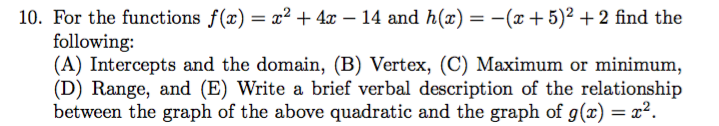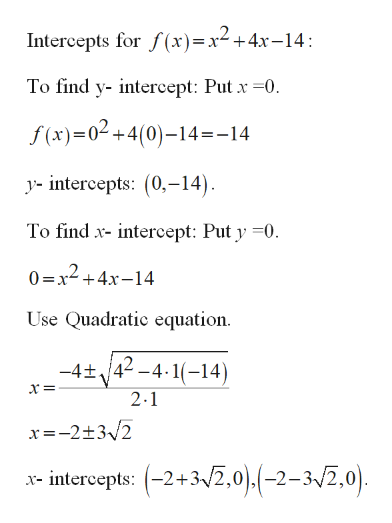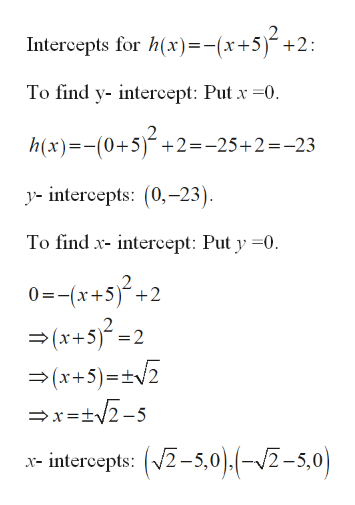# 10. For the functions f(x) = x² + 4x – 14 and h(x) = -(x +5)² +2 find thefollowing:(A) Intercepts and the domain, (B) Vertex, (C) Maximum or minimum,(D) Range, and (E) Write a brief verbal description of the relationshipbetween the graph of the above quadratic and the graph of g(x) = x².

Question
6 viewshelp_outlineImage Transcriptionclose10. For the functions f(x) = x² + 4x – 14 and h(x) = -(x +5)² +2 find the following: (A) Intercepts and the domain, (B) Vertex, (C) Maximum or minimum, (D) Range, and (E) Write a brief verbal description of the relationship between the graph of the above quadratic and the graph of g(x) = x². fullscreen
check_circle

Step 1

Since you have posted a question with multiple sub-parts, we will solve first three sub-parts for you. To get remaining sub-part solved please repost the complete question and mention the sub-parts to be solved.

Step 2

Part (A):

Find Intercept:

Consider f(x)=y.help_outlineImage TranscriptioncloseIntercepts for f(x)=x²+4x-14: To find y- intercept: Put x =0. f (x)=0² +4(0)–14=-14 y- intercepts: (0,-14). To find x- intercept: Put y =0. 0 =x² +4x-14 Use Quadratic equation. 42 -4·1(-14) -4+, 2.1 x=-2+3/2 x- intercepts: (-2+3/2,0),(-2-3/2,0). fullscreen
Step 3

Consider h(x)=y.

...help_outlineImage TranscriptioncloseIntercepts for h(x)=-(x+5)-+2: To find y- intercept: Put x =0. h(x)=-(0+5)´+2=-25+2=-23 %3D y- intercepts: (0,-23). To find x- intercept: Put y =0. 0=-(x+s}?+2 = (x+5}? =2 =(x+5)=+V2 =x=tV2-5 x- intercepts: (V2-5,0).(-/2-5,0) fullscreen

### Want to see the full answer?

See Solution

#### Want to see this answer and more?

Solutions are written by subject experts who are available 24/7. Questions are typically answered within 1 hour.*

See Solution
*Response times may vary by subject and question.
Tagged in

### Other